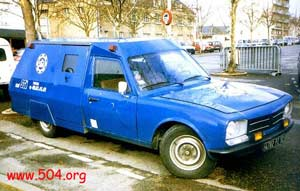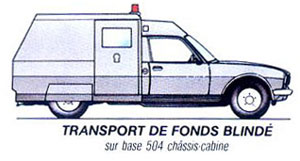## The image of the week!!

This week, I'd like you to discover a very special 504, perfect to transport your valuable objects... Yet another face for the 504!!No, no, your eyes don't lie to you! The 504 did even exist as an Amored Van to transport funds!! I knew that such a transformation was possible since I had found the drawing (placed under the photo) in a Peugeot Brochure on the 504 Pick-up. But this time, this is a real photo, proving that it really existed! Useless to tell you that I'd really like to have such a beast in my small 504 collection... Ok, it's not useful every day, I admit it, but I guess one might feel in complete security in that 504 model! This photo is scanned from the great book "La 504 de Mon Père" from François Allain.

index index index map map map index index index index index index index index index index index index index index index index index index index index index index index index index index index index index index index index index index index index index index index index index index index index index index index index index index index index index index index index index index index index index index index index index index index index index index index index index index index index index index index index index index index index index index index index index index index index index index index index index index index index index index index index index index index index index index index index index index index index index index index index index index index index index index index index index index index index index index index index index index index index index index index index index index index index index index index index index index index index index index index index index index index index index index index index index index index index index index index index index index index index index index index index index index index index index index index index index index index index index index index index index index index index index index index index index index index index index index index index index index index index index index index index index index index index index index index index index index index index index index index index index index index index index index index index index index index index index index index index index index index index index index index index index index index index index index index index index index index index index index index index index index index index index index index index index index index index index index index index index index index index index index index index index index index index index index index index index index index index index index index index index index index index index index index index index index index index index index index index index index index index index index index index index index index index index index index index index index index index index index index index index index index index index index index index index index index index index index index index index index index index index index index index index index index index index index index index index index index index index index index index index index index index index index index index index index index index index index index index index index index index index index index index index index index index index index index index index index index index index index index index index index index index index index index index index index index index index index index index index index index index index index index index index index index index index index index index index index index index index index index index index index index index index index index index index index index index index index index index index index index index index index index index index index index index index index index index index index index index index index index index index index index index index index index index index index index index index index index index index index index index